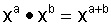## EXPONENTS ARE NOT ON THE LEVEL

"The Laws or Rules of Exponent Computation""Say That Again, Then Explain It" To Add number with the same base, the exponents must be equal.To Subtract numbers with the same base, the exponents must be equal.To Multiply numbers with the same base, copy the base and add the exponents.To Divide numbers with the same base, copy the base and subtract the exponents.To Raise to A Power copy the base, multiply the exponents.To Take A Root copy the base, divide the exponent by the index.To Add number with the same base, the exponents must be equal.To Subtract numbers with the same base, the exponents must
be equal.Example #1: Simplify: 3² + 3² 3² + 3² = 9 + 9 = 18 OR 3² + 3² = 1 · 3² + 1 · 3² = (1 + 1) · 3² = (2) · 3² = (2)(9) = 18 Note: The computation does not involve the exponents.

 Example #2: Simplify: x² + x² x² + x² = 1 · x² + 1 · x² = (1 + 1) · x² = (2) · x² = (2)(x²) = 2x²

 Example #3: Simplify: 3² + 3³ 3² + 3³ = 3² + 3³ Note: The exponents are not the same, but, here one may use arithmetic. 3² + 3³ = 9 + 27 = 36

 Example #4: Simplify: x² + x³ x² + x³ = x² + x³ Note: The exponents are not the same, and arithmetic can't help. x² + x³ = x² + x³

To Multiply numbers with the same base, copy the base andExample #1: Simplify: 3² · 3² 3² · 3² = 9 · 9 = 81 ORExample #2: Simplify: x² · x² x² · x² = x · x · x · x =ORExample #3: Simplify: 3² · 3³ 3² · 3³ = 9 · 27 = 243 ORExample #4: Simplify: x² · x³ORTo Divide numbers with the same base, copy the base and
subtract the exponents.Example #1: Simplify: 3² ÷ 3² 3² ÷ 3² = 9 ÷ 9 = 1 OR theseExample #2: Simplify: x² ÷ x²Example #3: Simplify: 3² ÷ 3³ 3² ÷ 3³ = 9 ÷ 27 = 9/27 = 1/3 ORORExample #4: Simplify: x² ÷ x³ORTo Raise to A Power copy the base, multiply the exponents.Example #1: Simplify: (3²)³ORExample #2: Simplify: (x²)³ORTo Take A Root copy the base, divide the exponent by the index.Example #1: Simplify:ORExample #2: Simplify:ORThis page is brought to you by MATHEMATICAL CONCEPTS, inc., publishers of MATH SPOKEN HERE!, ISBN: 0-9623593-5-1.

You are hereby granted permission to make ONE printed copy of this page and its picture(s) for your PERSONAL and not-for-profit use. YOU MAY NOT MAKE ANY ADDITIONAL COPIES OF THIS PAGE, ITS PICTURE(S), ITS SOUND CLIP(S), OR ITS ANIMATED GIFS WITHOUT PERMISSION FROM: classes@mathnstuff.com or by mail to the address below.### MATHEMATICAL CONCEPTS, inc.

85 First Street, Keyport, NJ 07735-1503     callus@mathnstuff.com   (732) 739-3951